## Electric charge can accumulate on an airplane in flight. You mayhave observed needle-shaped metal extensions on the wing tips andtail of an

Question

Electric charge can accumulate on an airplane in flight. You mayhave observed needle-shaped metal extensions on the wing tips andtail of an airplane. Their purpose is to allow charge to leak offbefore much of it accumulates. The electric field around the needleis much larger than the field around the body of the airplane and,can become large enough to produce dielectric breakdown of the air,discharging the airplane. To model this process, assume that twocharged spherical conductors are connected by a long conductingwire and a charge of 22.0 µC isplaced on the combination. One sphere, representing the body of theairplane, has a radius of 6.00 cm, and the other, representing thetip of the needle, has a radius of 2.00 cm.(a) What is the electric potential of eachsphere?(b) What is the electric field at the surface of each sphere?

in progress 0
5 months 2021-08-26T19:57:18+00:00 1 Answers 1 views 0

1. Answer: (a)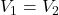= 24.75x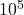V

(b)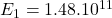V/m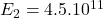V/m

Explanation: Eletric Potential (V) is the amount of energy necessary to move a charged particle inside an electric field. It is calculated as: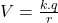where:

k is coulomb’s constant: k = 9.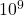N.m²/C²

q is the charge of the object

r is the distance

Electric Field (E) is what surrounds an electric particle in a way that every particle inside the field is influenciated by it, through force of attraction or of repulsion. When related to electric potential, can be calculated as: E =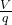a) The ratio of the two charges is proportional to the ratio of the two radii: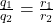where 1 represents the sphere of the body of the airplane and 2 is the tip of the needle.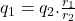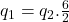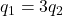(1)

The combine charges of spheres results in a charge of 22.0µC, which means: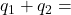22.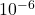Substitute and resolve: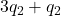= 22.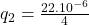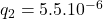C

Using (1) to find the other charge: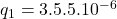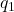= 16.5.C

Now, to determine electric potential for each sphere:

Electric Potential for Sphere 1: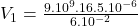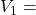24.75.V

Electric Potential for Sphere 2: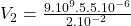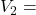24.75.V

The electric potential of each sphere is the same and has magnitude 24.75.V.

b) Electric Field for Sphere 1: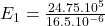V/m

Electric field for Sphere 2: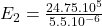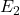=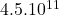V/m